﻿
﻿

## 三阶魔方-CFOP-PLL公式·技巧 PLL10(V) 手法详解

PLL情况：PLL10(V)

 示意图 实例图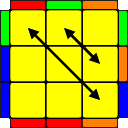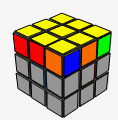详细图解：

1.正向：(R'UR') d'(R'F') (R2U'R'U) (R'FRF)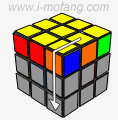→→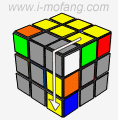→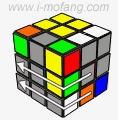R' U R' d' →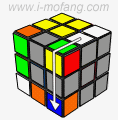→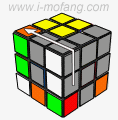→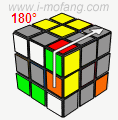→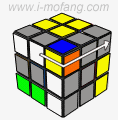R' F' R2 U' →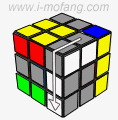→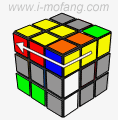→→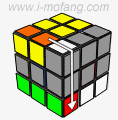R' U R' F →→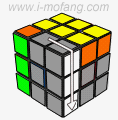→R F

 本站选用公式：顶(R' U R') d' 前(R' F') 底(R2 U' R' U) (R' F R) 顶F d’用左拇指和食指完成（类似做U'的感觉，U'做完之后，左手马上移到原来的右面，把原来的右面变为F面）。 【特征】 某直角的两边，各边上靠近直角的棱块角块颜色分别相同；其余两边的任一边上都没有两个颜色相同的块。 如图左边的两个相邻的蓝色，前面的两个相邻的红色。 【记忆】 其他参考公式： - -

2.逆向：R' U R' d' R' F' R2 U' R' U R' F R F U'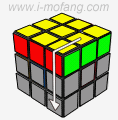→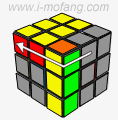→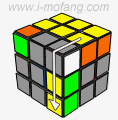→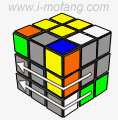R' U R' d' →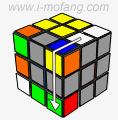→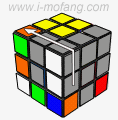→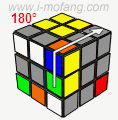→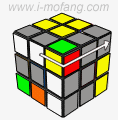R' F' R2 U' →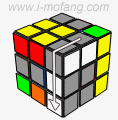→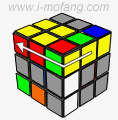→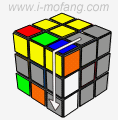→R' U R' F →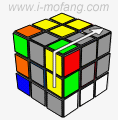→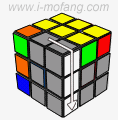→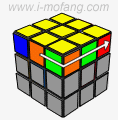→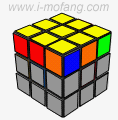R F U'

## ◆